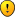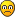## How many minimal sudokus has an average grid ?

Everything about Sudoku that doesn't fit in one of the other sections
coloin wrote:Well.....great work by Red Ed and Ocean - I can just about see how they are getting their results - very impressive !

from Ocean's data - it would appear that the average size minmal puzzle would appear to have 27 clues !

Hopefully we shall soon have confirmation regarding the number of puzzles per individual grid.

It seems that the method used will bias the distribution towards high number of clues. How much this bias is, depends on some currently unknown properties.

Meanwhile the sampling continues, and estimates for 30s (upper end) and 20s (lower end) now emerge. But will not report new numbers yet, because of the aforementioned uncertainties.

In addition to slow convergence, the numbers r and m converge to some fixed values different from the 'true mean', because of 'biased sampling'. If we could reliably estimate the error bounds, we could also calculate upper/lower bounds for M(C), the number of minimals with C clues.
Ocean

Posts: 442
Joined: 29 August 2005

JPF wrote:That's not the first time you take the liberty of giving (me) good or bad points without logical support, any rational in your answers or convincing arguments.
Could you tell me why ?
Calm down dear, it's only a commercial (as we say in this country)My 2.72 came from, oh, I dunno, a couple of hours processing @ 25 trials/sec; say 180K trials. Standard deviation of each estimate for 1/P using my "not the most obvious" method is ~.35, so s.d. of the mean is <.001, so 2 decimal places seems OK. But I wasn't being scientific, I was merely waiting until the thing looked stable-ish.

I've not bothered with confidence intervals because I found that I got different figures from different grid generators. If my generator was biased then it could be embarrassing to assert 95% confidence that 1/P lay in some interval when my generator might be converging to an answer that was some way off the mark. Hence, I am interested in building an unbiased grid generator. Thus, this is a helpful contribution:
m_b_metcalf wrote:"Mersenne Twister (MT) is a pseudorandom number generator ...

Anyway, I'm glad that my subgrid-sampling idea has generated so much discussion.
Red Ed

Posts: 633
Joined: 06 June 2005

Now, getting back to this ...
Ocean wrote:
Code: Select all
` Subgraph 2 (three levels): c=81           A ---               /  \   \                 /    \   \             /|\   /\   \ c=80       B C D I  J   L             |X X|  \ / c=79       E F G   K                  \|/              H`
...
How would you assess your method for finding minimals, given the cases above?
Let F(X,C) be an estimate (which is path-dependent) of the number of minimals at any level below node X, where X is at level C.

Here are the paths:
• ABEH. Hanging off node A, the algorithm sees 1 minimal and 5 non-minimals, so F(A,81) = (1+5*F(B,80)) / (82-81). Hanging off node B, the algorithm sees 0 minimals and 2 non-minimals, so F(B,80) = (0+2*F(E,79)) / (82-80). Hanging off node E, the algorithm sees 1 minimal and 0 non-minimals, so F(C,79) = (1+0) / (82-79). Putting these together, we get F(A,81) = 8/3.
• Paths ABFH, ACEH, ACGH, ADFH, ADGH give rise to the same estimate, 8/3.
• AIK. Hanging off node A, the algorithm sees 1 minimal and 5 non-minimals, so F(A,81) = (1+5*F(I,80)) / (82-81). Hanging off node I, the algorithm sees 1 minimal and 0 non-minimals, so F(I,80) = (1+0) / (82-80). Putting these together, we get F(A,81) = 7/2.
• Path AJK gives rise to the same estimate, 7/2.
• AL. This path can't occur, since the path searcher always branches down through a non-minimal node if that is possible.
The six paths down to H each occur with probability 1/5 * 1/2 = 1/10, so 60% of the time we'll get the 8/3 estimate for the number of minimals. The two paths down to K each occur with probability 1/5, so 40% of the time we'll get the 7/2 estimate.

Combining these, the estimated number of minimals is .6*8/3 + .4*7/2 = 3, as we hoped. ( Phew!)
Red Ed

Posts: 633
Joined: 06 June 2005

Red Ed wrote:Let F(X,C) be an estimate (which is path-dependent) of the number of minimals at any level below node X, where X is at level C.

Here are the paths:

Thanks for taking the time to illustrate the working of your algorithm!

Is in thinking modus right now. Hoping to improve a few things.
Ocean

Posts: 442
Joined: 29 August 2005

Ocean wrote:Hoping to improve a few things.
Improving convergence would be great if that's on your to-do list ... the current algorithm is far too slow.
Red Ed

Posts: 633
Joined: 06 June 2005

Meanwhile.........whist the thinking goes on.....

The mc grid - each puzzle has 648 isomorphic puzzles

If there are approx 2*10^15 puzzles with 27 clues

That would mean approx 3*10^12 non-isomorphic puzzles

Which means we should start to get duplicates at around 2.5 million puzzles - this is doable.......

Gsf's program i believe can generate [? randomly] the puzzles and canonicalize. It must do duplicates too.

C
coloin

Posts: 1864
Joined: 05 May 2005

coloin wrote:Gsf's program i believe can generate [? randomly] the puzzles and canonicalize. It must do duplicates too.

to this point I've tried to keep a small memory footprint, leaving dup checking
to external sort/uniq on the output puzzle stream

the current code has splay tree code for checking mutable clue isomorphism
this could be used to gather canonical puzzles from the generator
at a cost of ~98 bytes per canonical puzzle, compressible to ~32+#clues bytes

what kind of output are you looking for? just the dups? or a stream of non-dups?

edit: randomly? yes, using a 64 bit PRNG for a 2^64 cycle
edit^2: the search for all minimal puzzles algorithm (-m) is row order deterministic
gsf
2014 Supporter

Posts: 7306
Joined: 21 September 2005
Location: NJ USA

Ive been having a rethink on the point of this exercise, and I can see the problems infered by just the questions you ask regarding the output......

However just by generating a large number of puzzles from the mc grid [all with x clues] , reducing them to a canonical form, I doubt whether we can rely on a value of n when we get our first duplicate puzzle. The errors are compounded by the ^2 required to complete the estimation of the total number of puzzles [N] with x clues.

N~1/2 n^2 ...... I dont know of a statistical method to validate an average value of "n" for a greater number of puzzles.

Suffice to say if you generated 2.5 million puzzles, canonicalized them and then found only one duplicate......
Then this would add support to the estimation of N with 27 clues [2*10^15]

gsf wrote:... the search for all minimal puzzles algorithm (-m) is row order deterministic
.......................ah......... that is a bit of a drawback !

However.....suex9s program ! [It outputs [? randomly] minimal puzzles above a specified size from a single grid].

C
coloin

Posts: 1864
Joined: 05 May 2005

coloin wrote:
gsf wrote:... the search for all minimal puzzles algorithm (-m) is row order deterministic
.......................ah......... that is a bit of a drawback !

a drawback for this search across a large number of puzzles
but well suited to the much smaller expected amount for finding all
minimal puzzles for a given sudoku with one solution

there's a lot of leeway in the generation code
in case you get more ideas
gsf
2014 Supporter

Posts: 7306
Joined: 21 September 2005
Location: NJ USA

The question of generating unbiased random grids has been opened by Red Ed here .

in the following lines, "random" means implicitly "with an uniform distribution".

Let's assume that we have a perfect random number generator and a perfect random grid generator.

What about unbiased subgrids (puzzles) ?

1. General puzzles :
P = M and G
M is a random mask of 81 binary digits.
G is a random grid.
easy.

2. General Puzzles with c clues :
Pc = Mc and G
Mc is a random mask of 81 binary digits such that it has c 1-digits and (81-c) 0-digits
G is a random grid.
easy.

 : Note that in cases 1&2, the puzzles can have more than one solution [N(P)>1 or N(Pc)>1]
In order to get valid puzzles, loop on G, M or Mc until N(P)=1 or N(Pc)=1.

3. Minimal puzzles :
One way to create minimal puzzle is to apply this well known procedure, N(P) beeing the number of solutions of P :

0. Select a random grid G
1. P = G
2. select a random clue c in P
3. Pm = P – [c]
4. if N(Pm)= 1 --> P = Pm : go to 2
5. P is minimal

Assuming that G and c are each randomly picked in their sets, is it clear that the distribution of P is uniform ? (i.e. in the set of the minimal puzzles )

JPF
JPF
2017 Supporter

Posts: 3873
Joined: 06 December 2005
Location: Paris, France

I think that your methods 1 & 2 are unbiased if you make a slight modification: each attempt to generate a puzzle should start with a new G and a new M (or Mc). To see why just picking a new Mc (say) is bad, consider what happens if c=17 and G doesn't contain a 17-clue puzzleMethod 3 won't work unless every solution grid G contains the same number of minimal puzzles. At least for 2x2 grids, that's not the case. I don't have any better suggestions, though.Red Ed

Posts: 633
Joined: 06 June 2005

Red Ed wrote:I think that your methods 1 & 2 are unbiased if you make a slight modification: each attempt to generate a puzzle should start with a new G and a new M (or Mc)

It's what I had in mind.

Red Ed wrote:Method 3 won't work unless every solution grid G contains the same number of minimal puzzles. At least for 2x2 grids, that's not the case. I don't have any better suggestions, though.You are right, and it's not clear to me if with this algorithm two minimal puzzles with the same solution grid have the same probability to occur.

JPF
JPF
2017 Supporter

Posts: 3873
Joined: 06 December 2005
Location: Paris, France

Previous